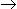# Chemical Engineering - Chemical Reaction Engineering

### Exercise :: Chemical Reaction Engineering - Section 3

1.

Space velocity

 A. describes the extensive operating characteristics of a tubular flow reactor. B. is the maximum feed rate pre unit volume of reactor for a given conversion. C. is a measure of the ease of the reaction. D. all (a), (b) and (c)

Explanation:

No answer description available for this question. Let us discuss.

2.

The rate of the chemical reaction AB doubles as the concentration of A i.e.., CA is doubled. If rate of reaction is proportional to CAn, then what is the value of n for this reaction ?

 A. 0.5 B. 1 C. 0 D. 2

Explanation:

No answer description available for this question. Let us discuss.

3.

A reaction which is catalysed by a base is catalysed by all substances which have a tendency to

 A. lose a proton B. gain a proton C. gain an electron D. none of these

Explanation:

No answer description available for this question. Let us discuss.

4.

For a homogeneous reaction of nth order, the dimension of the rate constant is given by

 A. l/(time)n B. (concentration)1 - n/(time) C. (concentration)n - 1/(time) D. none of these

Explanation:

No answer description available for this question. Let us discuss.

5.

The fractional volume change between no conversion and complete conversion, for the isothermal gas phase reaction, 2AR, is

 A. 0.5 B. -0.5 C. 1 D. 1.5